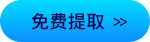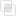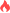python逆序输出三位数2021-04-25 10:18:14603浏览 · 0收藏 · 0评论1、整数法取余取整。

Python不像C语言那样自动截取保留整数，需要通过int进行转换。

```class Solution:
"""
@param number: A 3-digit number.
@return: Reversed number.
"""
def reverseInteger(self, number):
g = number%10         #取出个位数
s = (int(number/10))%10   #取出十位数
b = int(number/100)         #取出百位数
return g*100+s*10+b
if __name__ == "__main__":
so = Solution()
print (so.reverseInteger(123))```

2、使用栈

```def reverseInteger(self, number):
number=str(number)#模拟入栈
l=list(number)
result=""
while len(l)>0:
result+=l.pop()#模拟出栈
return int(result)```

3、依次表示输入的3位数百位数、10位数和10位数，交换100位数。

```#输入number = 123,输出321
number = int(input('请输入一个三位数：'))
a = number%10  #个位
b = number//10%10  #十位
c = number//100  #百位
re_number = a*100 + b*10 + c
print('%d的反转数是:%d'%(number,re_number)) #第一种输出方式
print('{0}的反转数是:{1}'.format(number,re_number)) #第二种输出方式```python怎么逆序Python中逆序输出字符串的六种方法数组怎样在python3中逆序输出?python 序列的逆序如何使用python中range()函数实现逆序遍历？算法：逆序对java反射调用指定的属性258

java获取class实例的方式636

java中反射提供哪些功能867